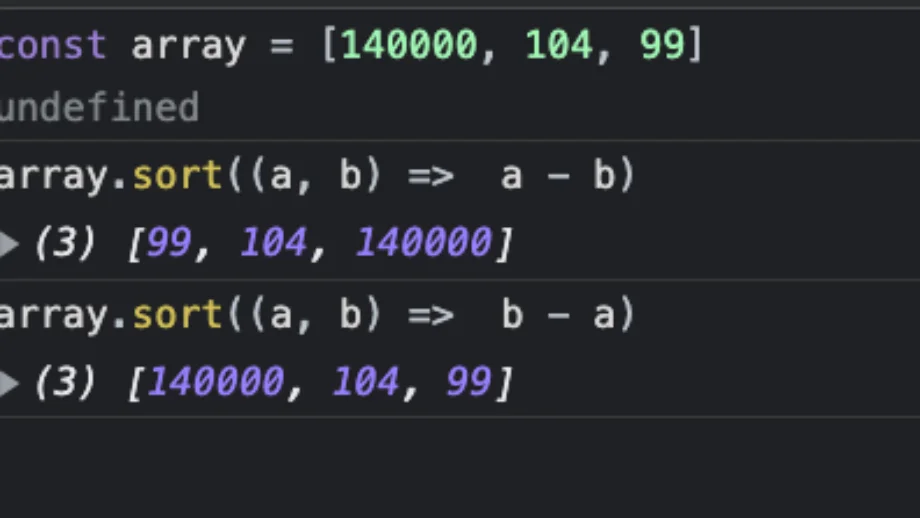# How to sort a Set in JavaScript

Share

ES6 introduced the set data structure in JavaScript. But sets are not ordered abstract data structures. So there is no .sort() property available on them. To sort a Set in JavaScript, we need to convert it into an array first.

Since arrays are sortable, we will then sort them, and then convert the array back to a Set after we are done. At some point, the spec might add a sort method to Set() that does all of this by default, but for now, there are no alternatives.

Here is what it looks like code-wise:

``````const numbers = new Set([5, 4, 1]);

const sortedNumbersArray = Array.from(numbers).sort((a, b) => a - b);

``````const strings = new Set(['x', 'z', 'y']);